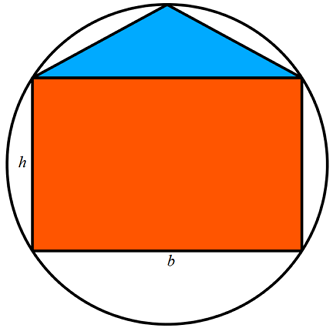# Rectangle And Triangle Inside Circle

Geometry Level 4Inscribe a rectangle of base $b$ and height $h$ and an isosceles triangle of base $b$ inside a circle of radius one as shown in the figure above.

The center of the circle coincides with the point of intersection of the diagonals of the rectangle.

For what value of $h$ do the rectangle and triangle have the same area ?

×